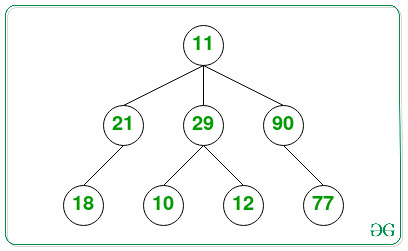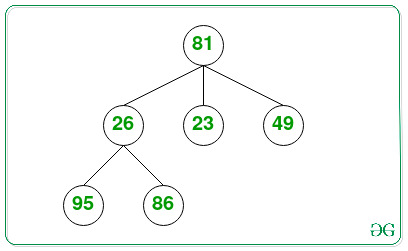Related Articles
Largest element in an N-ary Tree
• Difficulty Level : Expert
• Last Updated : 03 May, 2021

Given an N-ary tree consisting of N nodes, the task is to find the node having the largest value in the given N-ary Tree.

Examples:

Input:Output: 90
Explanation: The node with the largest value in the tree is 90.

Input:Output: 95
Explanation: The node with the largest value in the tree is 95.

Approach: The given problem can be solved by traversing the given N-ary tree and keeping track of the maximum value of nodes that occurred. After completing the traversal, print the maximum value obtained.

Below is the implementation of the above approach:

## C++

 `// C++ program for the above approach ` ` `  `#include ` `using` `namespace` `std; ` ` `  `// Structure of a ` `// node of N-ary tree ` `struct` `Node { ` `    ``int` `key; ` `    ``vector child; ` `}; ` ` `  `// Stores the node with largest value ` `Node* maximum = NULL; ` ` `  `// Function to create a new Node ` `Node* newNode(``int` `key) ` `{ ` `    ``Node* temp = ``new` `Node; ` `    ``temp->key = key; ` ` `  `    ``// Return the newly created node ` `    ``return` `temp; ` `} ` ` `  `// Function to find the node with ` `// largest value in N-ary tree ` `void` `findlargest(Node* root) ` `{ ` `    ``// Base Case ` `    ``if` `(root == NULL) ` `        ``return``; ` ` `  `    ``// If maximum is NULL, return ` `    ``// the value of root node ` `    ``if` `((maximum) == NULL) ` `        ``maximum = root; ` ` `  `    ``// If value of the root is greater ` `    ``// than maximum, update the maximum node ` `    ``else` `if` `(root->key > (maximum)->key) { ` `        ``maximum = root; ` `    ``} ` ` `  `    ``// Recursively call for all the ` `    ``// children of the root node ` `    ``for` `(``int` `i = 0; ` `         ``i < root->child.size(); i++) { ` `        ``findlargest(root->child[i]); ` `    ``} ` `} ` ` `  `// Driver Code ` `int` `main() ` `{ ` `    ``// Given N-ary tree ` `    ``Node* root = newNode(11); ` `    ``(root->child).push_back(newNode(21)); ` `    ``(root->child).push_back(newNode(29)); ` `    ``(root->child).push_back(newNode(90)); ` `    ``(root->child->child).push_back(newNode(18)); ` `    ``(root->child->child).push_back(newNode(10)); ` `    ``(root->child->child).push_back(newNode(12)); ` `    ``(root->child->child).push_back(newNode(77)); ` ` `  `    ``findlargest(root); ` ` `  `    ``// Print the largest value ` `    ``cout << maximum->key; ` ` `  `    ``return` `0; ` `} `

Output:

```90
```

Time Complexity: O(N)
Auxiliary Space: O(1)

My Personal Notes arrow_drop_up
Recommended Articles
Page :